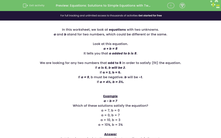# Equations: Solutions to Simple Equations with Two Unknowns

In this worksheet, students must find pairs of numbers that satisfy a simple equation with two unknowns.Key stage:  KS 2

Curriculum topic:   Algebra

Curriculum subtopic:   Use Equations

Popular topics:   Algebra worksheets

Difficulty level:#### Worksheet Overview

In this worksheet, we look at equations with two unknowns.

a and b stand for two numbers, which could be different or the same.

Look at this equation.

a + b = 8

It tells you that a added to b is 8.

We are looking for any two numbers that add to 8 in order to satisfy (fit) the equation.

If a is 6, b will be 2.

If a = 2, b = 6.

If a = 4½, b = 3½.

Example

a - b = 7

Which of these solutions satisfy the equation?

a = 7, b = 0

a = 0, b = 7

a = 10, b = 3

a = 10¾, b = 3¾

Put the given pairs into the equation to see if a - b is 7 or not.

 a = 7, b = 0 fits because 7 - 0 = 7 a = 0, b = 7 does not fit because 0 - 7 = -7 (not 7) a = 10, b = 3 fits because 10 - 3 = 7 a = 10¾, b = 3¾ fits because 10¾ - 3¾ = 7

### What is EdPlace?

We're your National Curriculum aligned online education content provider helping each child succeed in English, maths and science from year 1 to GCSE. With an EdPlace account you’ll be able to track and measure progress, helping each child achieve their best. We build confidence and attainment by personalising each child’s learning at a level that suits them.

Get started